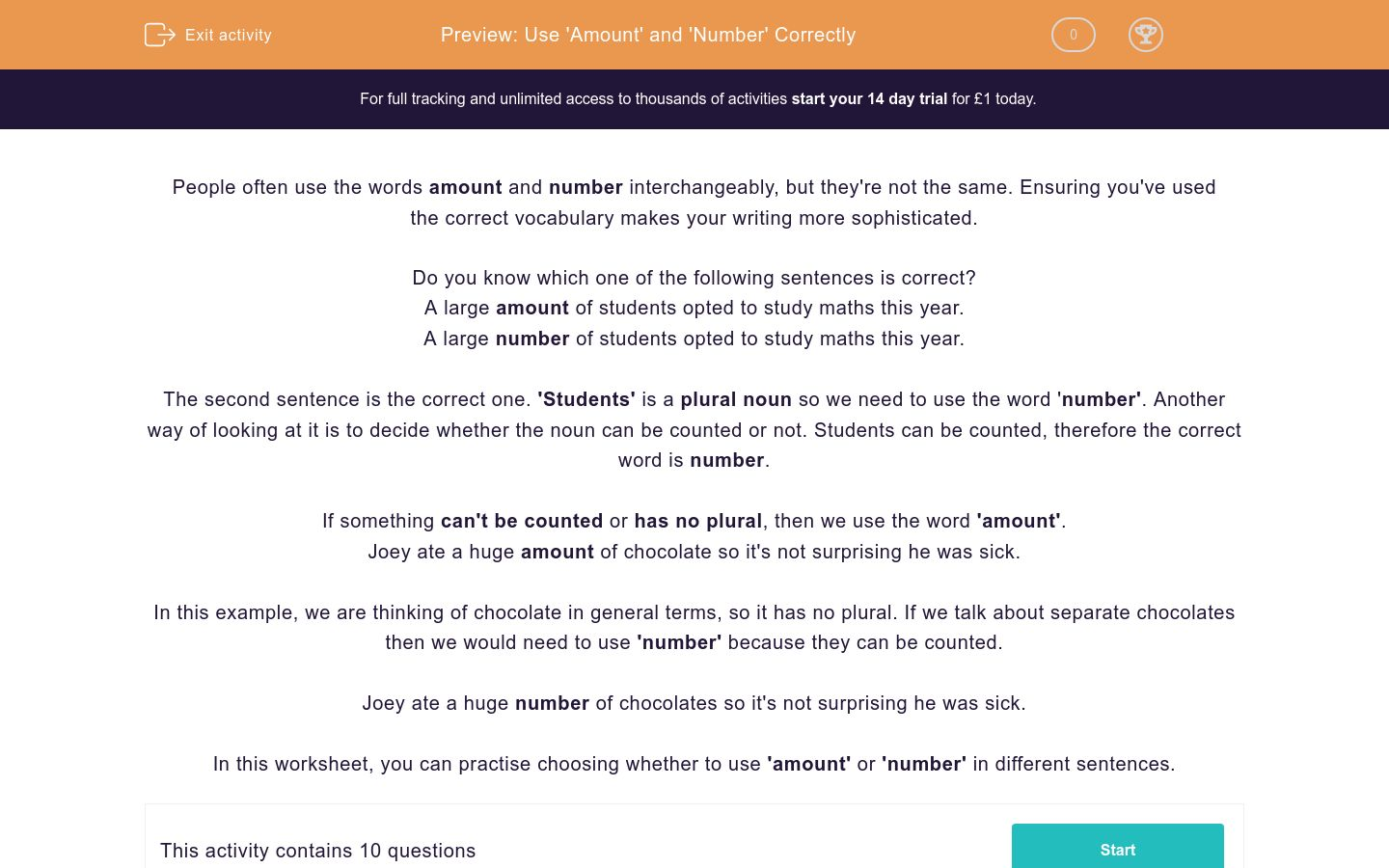# Use 'Amount' and 'Number' Correctly

In this worksheet, students practise using 'amount' and 'number' in the correct context.Key stage:  KS 3

Curriculum topic:   Writing

Curriculum subtopic:   Use Accurate Grammar, Punctuation and Spelling

Difficulty level:### QUESTION 1 of 10

People often use the words amount and number interchangeably, but they're not the same. Ensuring you've used the correct vocabulary makes your writing more sophisticated.

Do you know which one of the following sentences is correct?

A large amount of students opted to study maths this year.

A large number of students opted to study maths this year.

The second sentence is the correct one. 'Students' is a plural noun so we need to use the word 'number'. Another way of looking at it is to decide whether the noun can be counted or not. Students can be counted, therefore the correct word is number.

If something can't be counted or has no plural, then we use the word 'amount'.

Joey ate a huge amount of chocolate so it's not surprising he was sick.

In this example, we are thinking of chocolate in general terms, so it has no plural. If we talk about separate chocolates then we would need to use 'number' because they can be counted.

Joey ate a huge number of chocolates so it's not surprising he was sick.

In this worksheet, you can practise choosing whether to use 'amount' or 'number' in different sentences.

Choose the correct word to complete the following sentence.

Peter spent a huge ______ of time on his project.

amount

number

Choose the correct word to complete the following sentence.

amount

number

Choose the correct word to complete the following sentence.

It was a shame that such a small ______ of people turned up.

amount

number

Choose the correct word to complete the following sentence.

Sarah put a great ______ of effort into her performance.

amount

number

Look at the sentence below.

The amount of children with asthma is increasing.

Is this grammatically correct or would 'number' sound better?

The sentence is correct as it is as it is.

'The number of children with asthma is increasing' is correct.

Choose the correct word to complete the following sentence.

The sentence is correct as it is as it is.

'The number of children with asthma is increasing' is correct.

Choose the correct word to complete the following sentence.

The ship that sank was carrying a large ______ of containers.

amount

number

Choose the correct word to complete the following sentence.

The _______ of urban foxes is increasing.

amount

number

Choose the correct word to complete the following sentence.

amount

number

Choose the correct word to complete the following sentence.

I am shocked at the ______ of drivers who still use their mobiles at the wheel.

amount

number

• Question 1

Choose the correct word to complete the following sentence.

Peter spent a huge ______ of time on his project.

amount
EDDIE SAYS
'Time' is a singular noun, therefore we use 'amount'.
• Question 2

Choose the correct word to complete the following sentence.

EDDIE SAYS
This time the noun (hours) is plural and can be counted. Therefore we use 'number' in this instance!
• Question 3

Choose the correct word to complete the following sentence.

It was a shame that such a small ______ of people turned up.

number
EDDIE SAYS
People can be counted therefore 'number' is correct. Is this beginning to become less daunting?
• Question 4

Choose the correct word to complete the following sentence.

Sarah put a great ______ of effort into her performance.

amount
EDDIE SAYS
'Effort' is not a countable noun, therefore 'amount' is the correct choice.
• Question 5

Look at the sentence below.

The amount of children with asthma is increasing.

Is this grammatically correct or would 'number' sound better?

'The number of children with asthma is increasing' is correct.
EDDIE SAYS
Children can be counted, therefore we use 'number'.
• Question 6

Choose the correct word to complete the following sentence.

EDDIE SAYS
Oil cannot be counted, therefore we use the word 'amount'. You're making great progress, let's keep pushing.
• Question 7

Choose the correct word to complete the following sentence.

The ship that sank was carrying a large ______ of containers.

number
EDDIE SAYS
This time the correct choice is 'number' because containers can be counted.
• Question 8

Choose the correct word to complete the following sentence.

The _______ of urban foxes is increasing.

number
EDDIE SAYS
Foxes can be counted, so we use 'number'. You're a pro by now.
• Question 9

Choose the correct word to complete the following sentence.

EDDIE SAYS
We think of salt as a quantity rather than as separate grains, so the correct choice is 'amount'.
• Question 10

Choose the correct word to complete the following sentence.

I am shocked at the ______ of drivers who still use their mobiles at the wheel.

number
EDDIE SAYS
Drivers can be counted, so we use 'number'. Great work, you've completed another activity! How about attempting another one so you feel super confident?
---- OR ----

Sign up for a £1 trial so you can track and measure your child's progress on this activity.

### What is EdPlace?

We're your National Curriculum aligned online education content provider helping each child succeed in English, maths and science from year 1 to GCSE. With an EdPlace account you’ll be able to track and measure progress, helping each child achieve their best. We build confidence and attainment by personalising each child’s learning at a level that suits them.

Get started